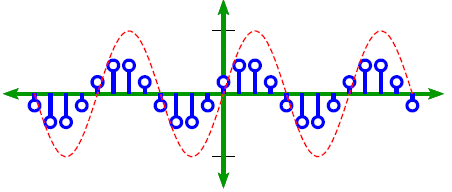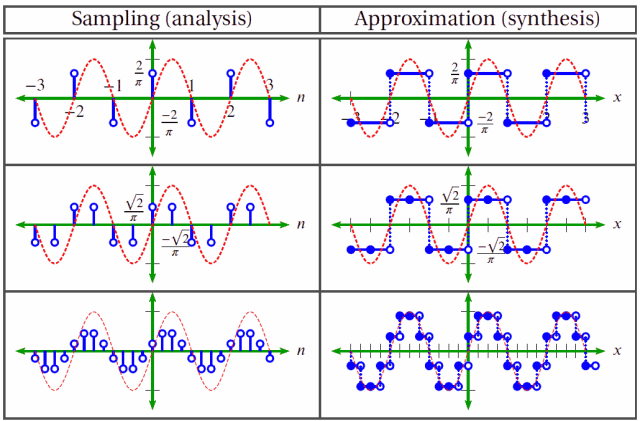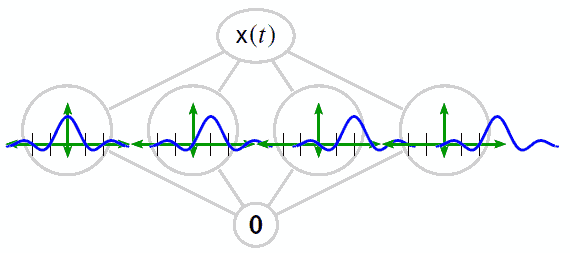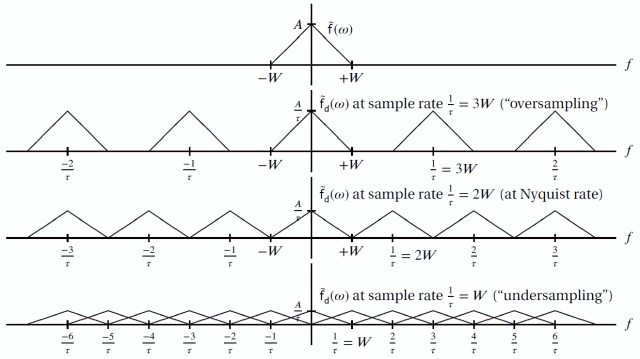# Sampling

June 2, 2021

Digital signal processing (DSP) in the real world often begins with sampling. Sampling takes measurements of a continuous waveform at regular intervals and generates a sequence of measured values.Sampling a sine waveform.

### Data Analysis and Sampling

Sampling is one of many ways to perform signal analysis. Like other analysis methods, it takes apart data. More specifically, it takes apart a continuous waveform into components or “samples.”

#### Data Synthesis and Sampling

After the data are separated into samples, is it possible to put them back together? That is, is synthesis possible? The answer is yes. This answer is one of the most surprising results in mathematics and is fundamental to DSP.According to the sampling theorem, if the sample rate is at least two times the highest frequency component of the sampled waveform, perfect reconstruction is possible.

Mathematically, the basis for a band-limited function x(t) is a series of sinc functions called a Cardinal series. The coefficients for the projection of x(t) onto the basis are the samples of x(t).#### Nyquist Frequency

The highest frequency component is called the Nyquist frequency. Sampling above this value is known as oversampling.

Undersampling occurs when the sample rate is less than two times this value. It can cause multiple copies of the signal in the frequency domain to overlap and result in aliasing. Aliasing occurs when a high-frequency signal masquerades as a low-frequency signal.